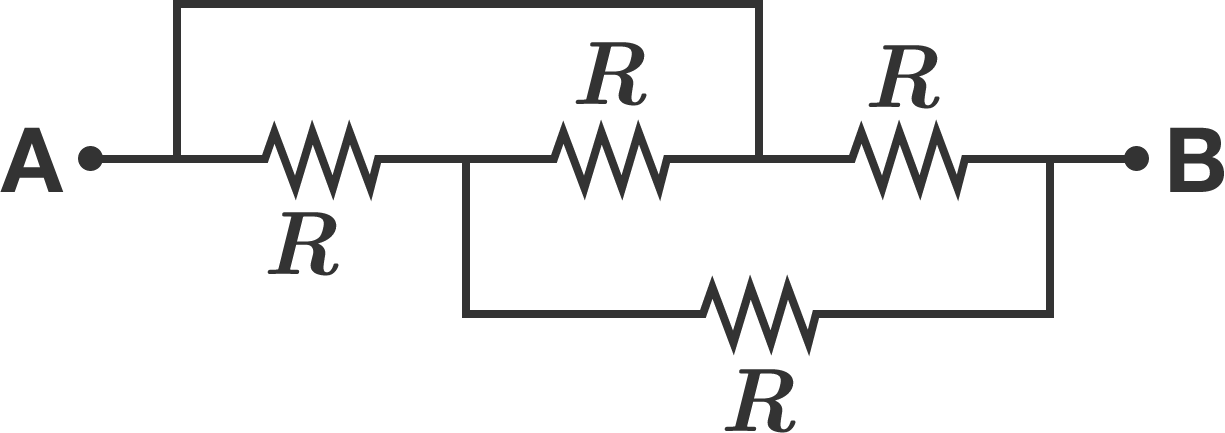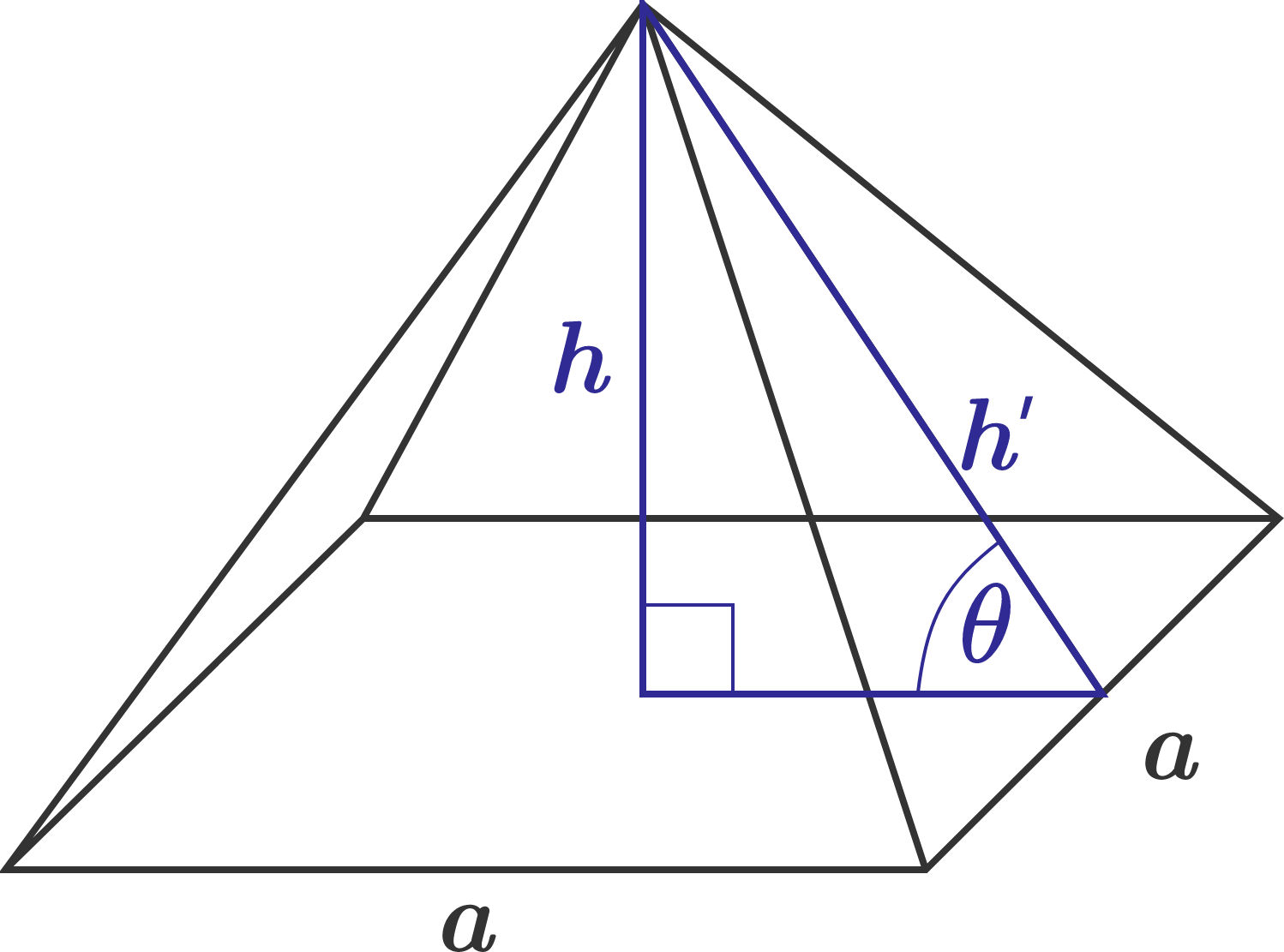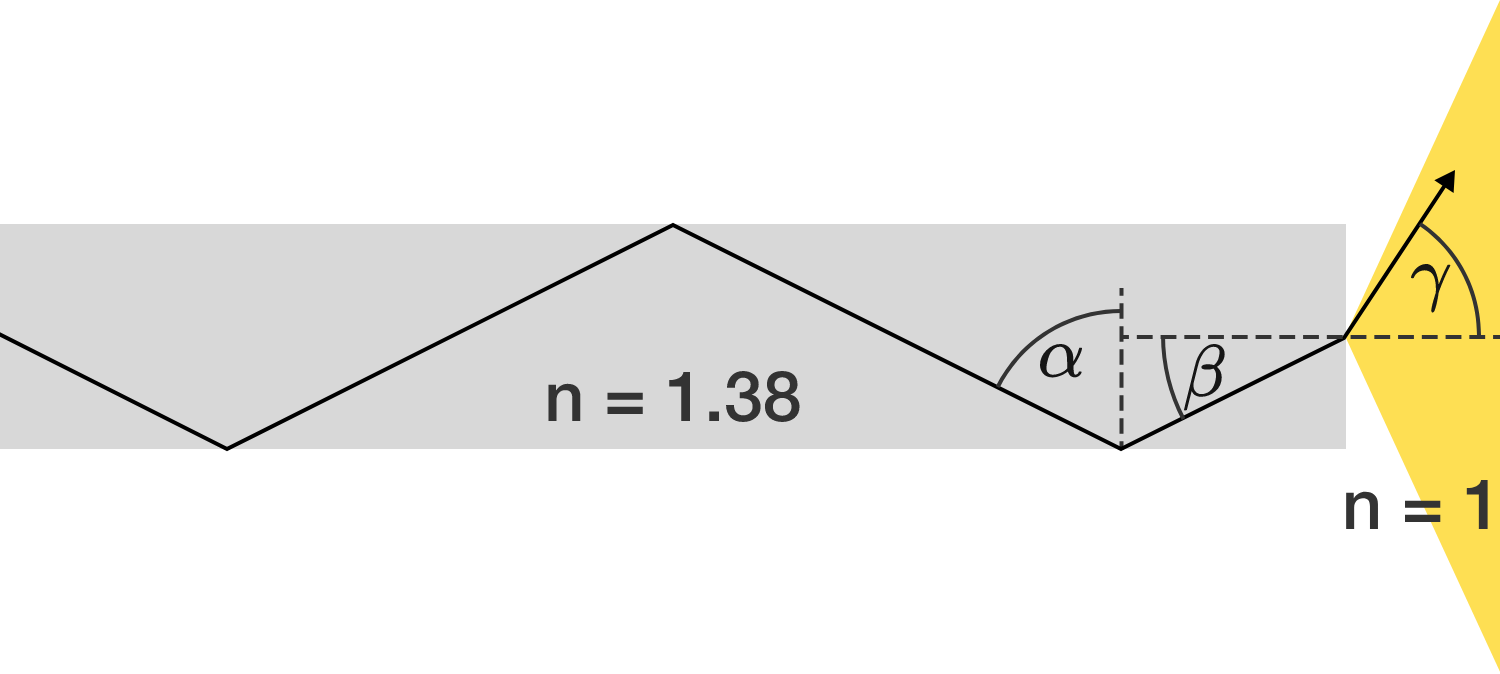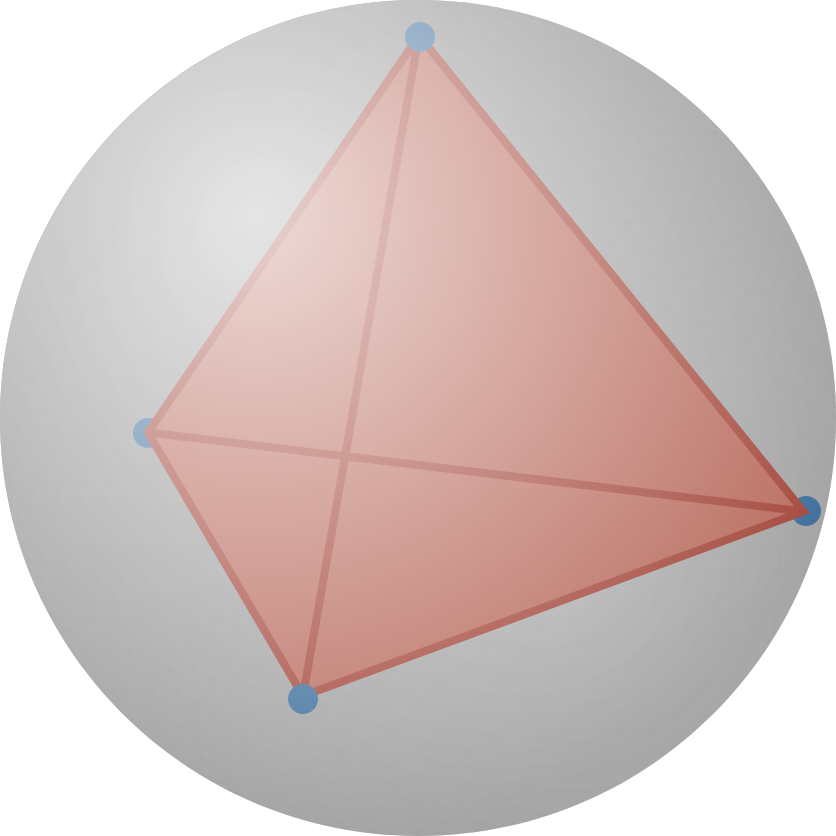# Problems of the Week

Contribute a problem

# 2017-10-23 Intermediate

What is the equivalent resistance between A and B?You are driving on a highway and are running out of gas. There are 3 upcoming gas stations. You need to stop at one of them, and you don't have the time to go backwards. However, the prices are different and randomly ordered (each of the 6 orderings is equally likely), and you don't know which will be the cheapest! You are only able to see each gas station's price if you stop at the station or pass by it.

You employ the strategy that maximizes your chance of picking the cheapest gas station. What is the probability that you pick the most expensive gas station?The Pharaoh would like to cover the lateral sides of his square pyramid with the finest marble. In order to save the cost of construction, the builder tries to reduce the exposed surface area of the pyramid as much as possible.

What is the angle of inclination $\theta$ (in degrees) that minimizes the exposed surface area given a constant volume of the pyramid?

Glass-fiber-guided light beams are continuously reflected at the interface between glass and air. How large is the maximum angle $\gamma$ (half opening angle) of the exiting light at the end of the glass fiber, in degrees, to the nearest integer?

The glass has a refractive index of $n = 1.38,$ while the air has an index of $n = 1.$Tom has a set of particles that repel each other with a force of $F(r)$ whose strength is proportional to $\frac{1}{r^2},$ where $r$ is the distance of separation between any pair of particles.

If Tom puts 8 of these particles into a rigid, frictionless sphere and allows them to come to rest, they'll align to the vertices of a certain $n$-faced polyhedron.

What is $n?$

For example, if Tom puts 4 of the particles into the sphere, they'll come to rest at the vertices of a regular tetrahedron, as shown.

×